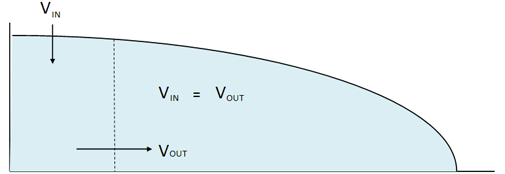# Dating by forward and inverse modelling

Computer models can be used to estimate the age along an ice core. The models describe the flow of the ice by taking into account the annual amount of snow in the past and the thinning of the annual layers as they are being compressed by the weight of the snow and ice above. Modelling of timescales is especially useful when the annual layers are too thin to be counted or when an approximate age model is needed.

In the central Antarctic ice cores where the annual layering is non-existent or unreliable due to extremely low accumulation rates, timescale modelling is often the only option, while most Greenland cores to some extent can be dated by annual layer counting. Timescale modelling is in this case used to obtain a preliminary timescale before the ice core has been drilled and analysed, and for dating the deeper parts of the ice where the annual layers get too thin to be safely identified.

## Timescale modelling basics

By making some simple assumptions about how the ice deforms under pressure in the interior of the ice sheet, it is possible to describe the deformation speed and velocity of the ice flow within the ice sheet reasonably correct. It is then possible to use the modelled velocities to predict the current depth of a snow particle which fell on the ice surface in the past. This analysis can be performed on the entire ice core hereby creating a depth-age relationship.

When we have a model that describes how the ice deforms, it is possible to calculate a depth-age relationship (usually referred to as an ice core timescale) by assuming that we know the amount of snowfall and melting at the surface of the ice sheet, and any melting taking place at bedrock (the so-called surface and basal mass balances). This is called forward modelling since we can calculate the timescale directly from the model parameters (in this case the surface and basal mass balances).

Especially the surface mass balance is not constant over time (the snow accumulation was for example less than half the present day values during the glacial), and the flow velocities will therefore also change over time, making the modelling problem more challenging.

## Forward and inverse modelling

As a further complication, it can be very hard to provide good estimates of the model parameters upon which the forward model is based, for example because especially the basal mass balance (the melt-off from underneath the ice sheet) is very hard to measure. This implies that a direct (forward) determination of a timescale is not always possible. However, often some other information on the depth-age relationship is available, e.g. because the age of certain layers are known from other dated records, or because annual layers can be counted in a part of the ice core. In this case, it is necessary to solve the inverse problem, where the objective is to determine the timescale as well as the unknown model parameters (e.g. surface and basal mass balance) from the known information. This is known as inverse modelling. The idea behind inverse modelling is to estimate which values the unknown model parameters are likely to have when we require that the timescale resulting from these parameters matches the data that we do have. One can say that we know parts of the result and want to construct a model that matches these results and then can be used to predict what we do not know.

The ice core science outreach webpage www.isarkiv.dk introduces a simple ice core model that can be used for ice core dating (currently in Danish only). The modelling section is the last part of the first case.